• matlab机器人工具箱的使用（2）
2022-04-10 14:40:58

# 学习目标：

matlab机器人工具箱的使用（2）

# 学习内容：

1、 工具箱常用函数 （旋转--旋转矩阵，旋转--变换矩阵，位移--变换矩阵，旋转矩阵--变换矩阵）

# 学习记录：

## 1.旋转–旋转矩阵

• rotx()------------x轴
• roty()------------y轴
• rotz()------------z轴

传入角度会得到旋转矩阵。

• eul2r()
传入欧拉角得到旋转矩阵
• tr2eul()
传入旋转矩阵得到欧拉角
r1=eul2r(90,60,30);

r2=rotz(90)*roty(60)*rotz(30);


***这里是绕当前坐标系旋转，所以是右乘

• rpy2r()
传入rpy角得到旋转矩阵
• tr2rpy()
传入旋转矩阵得到rpy角
r1=rpy2r(90,60,30);

r2=rotz(30)*roty(60)*rotx(90);


***1.rpy分别是横滚-俯仰-航偏角
2.这里是绕之前坐标系旋转，所以是左乘

看一下代码跑出了结果：

>> r1=eul2r(90,60,30);

r2=rotz(90)*roty(60)*rotz(30);
>> r1

r1 =

0.9491    0.2837    0.1366
0.3114   -0.9104   -0.2725
0.0470    0.3012   -0.9524

>> r2

r2 =

0.9491    0.2837    0.1366
0.3114   -0.9104   -0.2725
0.0470    0.3012   -0.9524


>> r1=rpy2r(90,60,30);

r2=rotz(30)*roty(60)*rotx(90);
>> r1

r1 =

-0.1469   -0.4847   -0.8622
0.9410    0.2001   -0.2728
0.3048   -0.8515    0.4268

>> r2

r2 =

-0.1469   -0.4847   -0.8622
0.9410    0.2001   -0.2728
0.3048   -0.8515    0.4268

>>


可以看出结果相同。

## 2.旋转–变换矩阵

• trotx()------------x轴
• troty()------------y轴
• trotz()------------z轴

传入角度会得到变换矩阵。

• eul2tr()
传入欧拉角得到变换矩阵
• tr2eul()
传入变换矩阵得到欧拉角
T1=eul2tr(90,60,30);

T2=trotz(90)*troty(60)*trotz(30);


***这里是绕当前坐标系旋转，所以是右乘

• rpy2tr()
传入rpy角得到变换矩阵
• tr2rpy()
传入旋转矩阵得到rpy角
T3=rpy2tr(90,60,30);

T4=trotz(30)*troty(60)*trotx(90);


***1.rpy分别是横滚-俯仰-航偏角
2.这里是绕之前坐标系旋转，所以是左乘

看一下代码跑出了结果：

>> T1=eul2tr(90,60,30);

T2=trotz(90)*troty(60)*trotz(30);
>> T1

T1 =

0.9491    0.2837    0.1366         0
0.3114   -0.9104   -0.2725         0
0.0470    0.3012   -0.9524         0
0         0         0    1.0000

>> T2

T2 =

0.9491    0.2837    0.1366         0
0.3114   -0.9104   -0.2725         0
0.0470    0.3012   -0.9524         0
0         0         0    1.0000

>>


>> T3=rpy2tr(90,60,30);

T4=trotz(30)*troty(60)*trotx(90);
>> T3

T3 =

-0.1469   -0.4847   -0.8622         0
0.9410    0.2001   -0.2728         0
0.3048   -0.8515    0.4268         0
0         0         0    1.0000

>> T4

T4 =

-0.1469   -0.4847   -0.8622         0
0.9410    0.2001   -0.2728         0
0.3048   -0.8515    0.4268         0
0         0         0    1.0000



可以看出结果相同。

• transl()

传入角度会得到变换矩阵。

## 4.旋转矩阵–变换矩阵

• t2r()
• r2t()
T=transl(1.5,1,0.5)*trotx(30)*trotz(60);
P=transl(T);
R=t2r(T);

>> T=transl(1.5,1,0.5)*trotx(30)*trotz(60);
P=transl(T);
R=t2r(T);
>> T

T =

-0.9524    0.3048         0    1.5000
-0.0470   -0.1469    0.9880    1.0000
0.3012    0.9410    0.1543    0.5000
0         0         0    1.0000

>> P
# 位移
P =

1.5000
1.0000
0.5000

>> R
# 旋转矩阵
R =

-0.9524    0.3048         0
-0.0470   -0.1469    0.9880
0.3012    0.9410    0.1543

>>

更多相关内容
• matlab机器人工具箱实现机械臂直线轨迹&圆弧轨迹规划（带sw模型+matlab程序+报告）
• 来源于课程设计，利用机器人工具箱10.4实现了关节角度空间轨迹生成和规划和笛卡尔空间圆弧轨迹和直线轨迹的生成规划。每种都提供了四种规划方式包含：匀速运动，带抛物线过渡段的轨迹规划，三次多项式轨迹规划，五次...
• 由于一个机器人包含的技术和任务太多，所以我们为了在之前的理论技术上开发新的算法就需要借助matlab工具箱来帮我们提高效率。MATLAB机器人工具箱就为了研究机器人的运动学、动力学及视觉等提供了一种方便
• Matlab机器人工具箱的讲解文档，作者就是工具箱的开发者，具体讲解了机器人视觉和机器人控制等问题。高清的文字版，并且带有书签和彩图。
• 非常好用的MATLAB机器人工具箱，大家喜欢的可以下载一下
• 仿真六自由度机器人运动学，工作空间，可以进行正逆运动学的运算，根据各机械臂的尺寸仿真工作空间
• 我的大三的机器人控制原理课程设计及，利用机器人工具箱，进行六自由度的机械臂的直线轨迹规划和圆弧轨迹规划，完全可用，参数可改，有注释说明
• matlab机器人工具箱最新版10.3.1，已经适用matlab2018a
• 该工具箱为MATLAB机器人工具箱，有PUMA560等多种常见的机器人模型，也可以自己定义模型，自己设定姿态变换，运动轨迹等，本资源只为更多的人共享，故设为最少的1分
• 机器人工具箱10.1版，有例子可以学习，希望对大家有所帮助！
• 文档详细介绍了如何运用MATLAB机器人工具箱对机器人进行运动和动力学分析
• 资源的内部包含MATLAB的9.10版本的机器人工具箱，及用户手册，使用说明等文档，详细说明见相关博文。
• 使用MATLAB:registered:机器人工具箱MATLAB:registered:中设计和开发2连杆机械臂 介绍： 这是我在Matlab中完成的一项业余项目。 该项目的目的是为了学习有关使用MATLAB:registered:的机器人技术的更多信息。 在一...

MATLAB机器人工具箱（一）

# 前言：

这里记录一下我的学习和使用过程，以及遇到的坑。我的版本是R2018a。

# 一、添加机器人工具箱——RTB工具箱：

方法一：
1.下载rvctools文件包，放在一个自己知道的目录下(任意路径都是可以的，但是为了方便管理，一般都安装在toolbox)，然后“设置路径”里面添加并保存。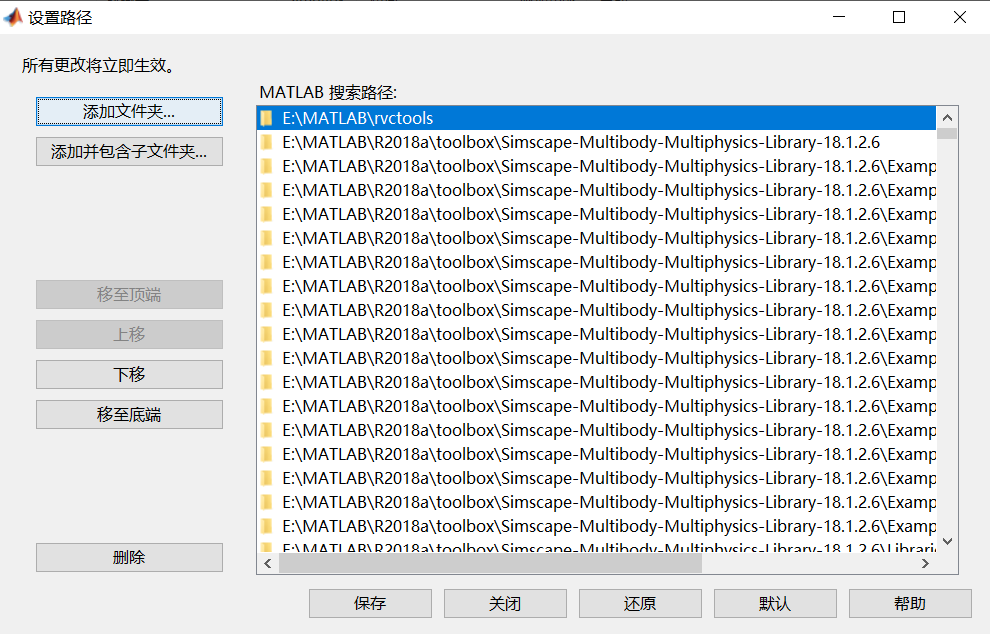2.在命令中输入statrup_rvc，之后会看到机器人工具箱启动成功。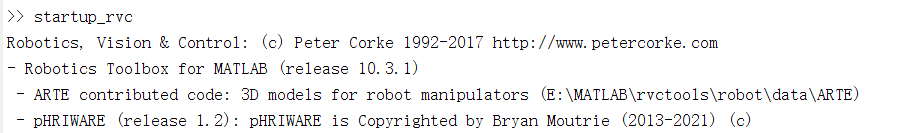3.最后在命令行中输入rtbdemo命令，会跳出一个操作界面。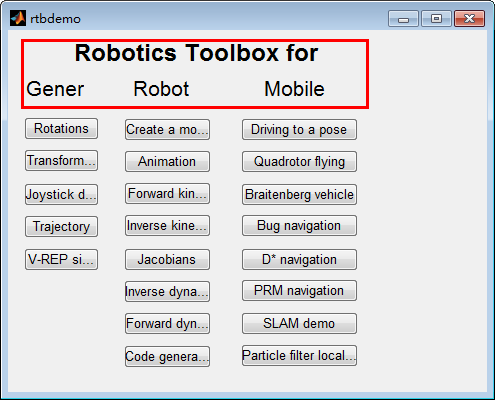但是这么做也有一个问题，就是每次打开都要重新通过statrup_rvc命令运行rvctools文件包，目前还没找到好的解决方法，欢迎大家提点。
如图所示：左边一列是通用函数的例子（如：旋转，平移，轨迹等）；中间主要是机械臂的基础函数，可以快速构建一个机器人；右边为移动机器人的一些历程。大家可以自己试试看。

方法二：
这里我看到别人直接下载RTB工具箱（注意版本号）并安装，然后输入rbtdemo命令运行，但我试了不太行，大家也可以试试看。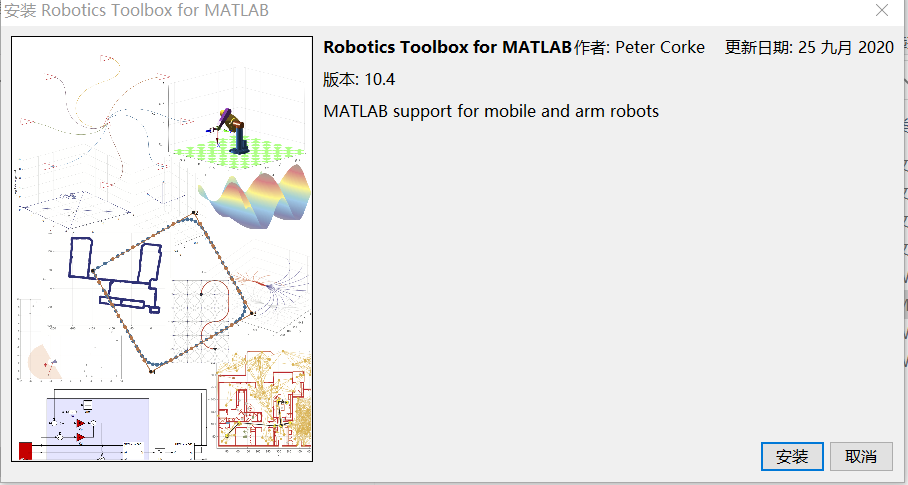# 1.“错误使用 eval，未定义…”：

在导入多体物理库时通过“设置路径”添加了toolbox里面的“Simscape-Multibody-Contact-Forces-Library-18.1.4.1”，结果就出现了如下错误：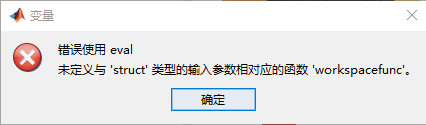甚至有的人安装好Matlab以后，什么操作也不做，仅仅是将软件打开就会有这样的报错。

解决方法一：（很多人用这种方法奏效了）
但对我来说似乎没什么用。

解决方法二：

>> addpath E:\MATLAB\R2018a\toolbox

%输入工具箱名称，此时一般会返回该工具箱的说明，也就是mathmodl
>> help mathmodl路径下content.m中的内容
%在命令行中输入如下，此时会返回mathmodl路径下所有的文件
>> what mathmodl
%再到mathmodl中随便找一个不与Matlab中重名的函数，比如xxx.m，在命令行中输入
>> which xxx.m
E:\MATLAB\rvctools\xxx.m


# 2.Matlab启动过慢：

这绝对是困扰我很久的一个问题，一度让我怀疑自己设备有问题（虽然确实慢）。
如果你的matlab停留在“正在初始化…”这个界面长达几分钟甚至更久，试试下面的方法（据说是最近几代新版本的共性问题）：
剩下的文件如图：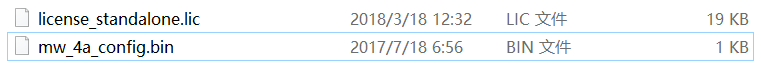然后速度瞬间提升了hhh。

# 3.Matlab启动的时候给出警告：

Warning: MATLAB Toolbox Path Cache is out of date and is not being used. Type 'help toolbox_path_cache' for more info.To get started, select MATLAB Help or Demos from the Help menu.

这个问题式因为安装工具箱之后没有更新缓存，解决方法：
File——>Preferences——>General——>选中enable toolbox path cache——>点击updata toolbox path cache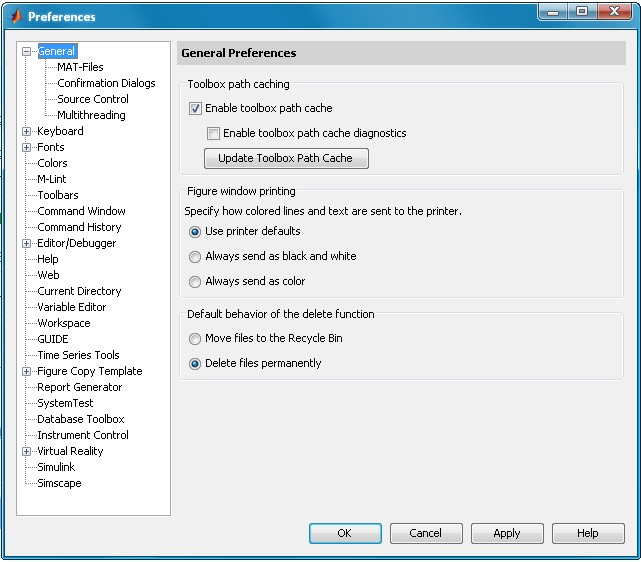# 1.复现双足机器人：

原博：JianRobSim%% 主代码
%% 说明：我用了原博的代码腿的位置不对，troty(-pi/2)改成了troty(pi/2)对了
%% creat walking robot model
clear all
%leg length
L1=0.15;L2=0.25;
%form a leg
leg=SerialLink([0, 0, 0, pi/2; 0, 0, L1, 0; 0, 0, -L2, 0 ],...
'name', 'leg', 'base', eye(4,4),'tool', ...
troty(pi/2)*trotx(-pi/2)*trotz(-pi/2),'offset', [pi/2  0  -pi/2]);
%% diplay the leg
%body wide and length
W = 0.2; L = 0.2;
%form a body
legs(1) = SerialLink(leg, 'name', 'leg1','base', transl(0, 0, -0.05)*trotz(pi/2));  %*trotz(pi/2)
legs(2) = SerialLink(leg, 'name', '.', 'base', transl(0, -W, -0.05)*trotz(pi/2));
% create a fixed size axis for the robot, and set z positive downward
clf;
axis([-0.3 0.25 -0.6 0.4 -0.19 0.45]); set(gca,'Zdir', 'reverse')
hold on
%plot body
plotcube([0.1 0.2 -0.12],[ -0.05  -0.2  0],1,[1 1 0]);
%% simulate moving
for i=0.01:0.02:0.4
end

% 身体控制器
function plotcube(varargin)
% PLOTCUBE - Display a 3D-cube in the current axes
%
%   PLOTCUBE(EDGES,ORIGIN,ALPHA,COLOR) displays a 3D-cube in the current axes
%   with the following properties:
%   * EDGES : 3-elements vector that defines the length of cube edges
%   * ORIGIN: 3-elements vector that defines the start point of the cube
%   * ALPHA : scalar that defines the transparency of the cube faces (from 0
%             to 1)
%   * COLOR : 3-elements vector that defines the faces color of the cube
%
% Example:
%   >> plotcube([5 5 5],[ 2  2  2],.8,[1 0 0]);
%   >> plotcube([5 5 5],[10 10 10],.8,[0 1 0]);
%   >> plotcube([5 5 5],[20 20 20],.8,[0 0 1]);
%% plotcube函数 长宽高  三维空间起点  颜色属性
% Default input arguments
inArgs = { ...
[10 56 100] , ... % Default edge sizes (x,y and z)
[10 10  10] , ... % Default coordinates of the origin point of the cube
.7          , ... % Default alpha value for the cube's faces
[1 0 0]       ... % Default Color for the cube
};

% Replace default input arguments by input values
inArgs(1:nargin) = varargin;

% Create all variables
[edges,origin,alpha,clr] = deal(inArgs{:});

XYZ = { ...
[0 0 0 0]  [0 0 1 1]  [0 1 1 0] ; ...
[1 1 1 1]  [0 0 1 1]  [0 1 1 0] ; ...
[0 1 1 0]  [0 0 0 0]  [0 0 1 1] ; ...
[0 1 1 0]  [1 1 1 1]  [0 0 1 1] ; ...
[0 1 1 0]  [0 0 1 1]  [0 0 0 0] ; ...
[0 1 1 0]  [0 0 1 1]  [1 1 1 1]   ...
};

XYZ = mat2cell(...
cellfun( @(x,y,z) x*y+z , ...
XYZ , ...
repmat(mat2cell(edges,1,[1 1 1]),6,1) , ...
repmat(mat2cell(origin,1,[1 1 1]),6,1) , ...
'UniformOutput',false), ...
6,[1 1 1]);

cellfun(@patch,XYZ{1},XYZ{2},XYZ{3},...
repmat({clr},6,1),...
repmat({'FaceAlpha'},6,1),...
repmat({alpha},6,1)...
);

% view(3);


在这个项目的最后，博主贴了一个simscape的仿真结果，确实流畅度高了很多。所以我的下一步学习计划就是simscape~# 2.复现机械臂：

参考博主，具体讲解可以点进去看。

clear ; clc; close all;
% 机器人各连杆DH参数
d1 = 0;
d2 = 86;
d3 = -92;

% 由于关节4为移动关节，故d4为变量，theta4为常量
theta4 = 0;
a1 = 400;
a2 = 250;
a3 = 0;
a4 = 0;

alpha1 = 0 / 180 * pi;
alpha2 = 0 / 180 * pi;
alpha3 = 180 / 180 * pi;
alpha4 = 0 / 180 * pi;

% 定义各个连杆，默认为转动关节
%           theta      d        a        alpha
L(1)=Link([  0         d1      a1      alpha1]); L(1).qlim=[-pi,pi];
L(2)=Link([  0         d2      a2      alpha2]); L(2).qlim=[-pi,pi]; L(2).offset=pi/2;
L(3)=Link([  0         d3      a3      alpha3]); L(3).qlim=[-pi,pi];

% 移动关节需要特别指定关节类型--jointtype
L(4)=Link([theta4       0      a4      alpha4]); L(4).qlim=[0,180]; L(4).jointtype='P';

% 把上述连杆“串起来”

% 定义机器人基坐标和工具坐标的变换
Scara.base = transl(0 ,0 ,305);
Scara.tool = transl(0 ,0 ,100);
Scara.teach();   %机器人示教界面

% 绘制动画
figure
joint(: , 1) = linspace(pi/6,pi/2,100);
joint(: , 2) = linspace(0,pi/4,100);
joint(: , 3) = linspace(pi/3,pi/2,100);
joint(: , 4) = linspace(0,160,100);
filename = 'demo.gif';
for i = 1:length(joint)
pause(0.01)
Scara.plot(joint(i,:));
f = getframe(gcf);
imind = frame2im(f);
[imind,cm] = rgb2ind(imind,256);
if i == 1
imwrite(imind,cm,filename,'gif', 'Loopcount',inf,'DelayTime',0.1);
else      imwrite(imind,cm,filename,'gif','WriteMode','append','DelayTime',0.1);
end
end


接下来有一个改进DH方法的代码，其实主要是 'modified’参数的改变：

clear ; clc; close all;
% 机器人各连杆参数值
d1 = 670;
d2 = 0;
d3 = 0;
d4 = 1280;
d5 = 0;
d6 = 215;

a1 = 0;
a2 = 312;
a3 = 1075;
a4 = 225;
a5 = 0;
a6 = 0;

alpha1 = 0 / 180 * pi;
alpha2 = -90 / 180 * pi;
alpha3 = 0 / 180 * pi;
alpha4 = -90 / 180 * pi;
alpha5 = 90 / 180 * pi;
alpha6 = -90 / 180 * pi;
% 建立连杆DH参数（修正的DH）
L(1)=Link([0 d1 a1 alpha1], 'modified'); L(1).qlim=[-pi,pi];
L(2)=Link([0 d2 a2 alpha2], 'modified');  L(2).qlim=[-pi,pi];L(2).offset = -pi/2;
L(3)=Link([0 d3 a3 alpha3], 'modified'); L(3).qlim=[-pi,pi];
L(4)=Link([0 d4 a4 alpha4], 'modified'); L(4).qlim=[-pi,pi];
L(5)=Link([0 d5 a5 alpha5], 'modified'); L(5).qlim=[-pi,pi];
L(6)=Link([0 d6 a6 alpha6], 'modified'); L(6).qlim=[-pi,pi];
% 定义机器人
FANUC.tool = transl(0,0,100);
FANUC.teach();


为了搞清楚standard DH和modified DH，我看了好久的资料，简单谈一下我的理解。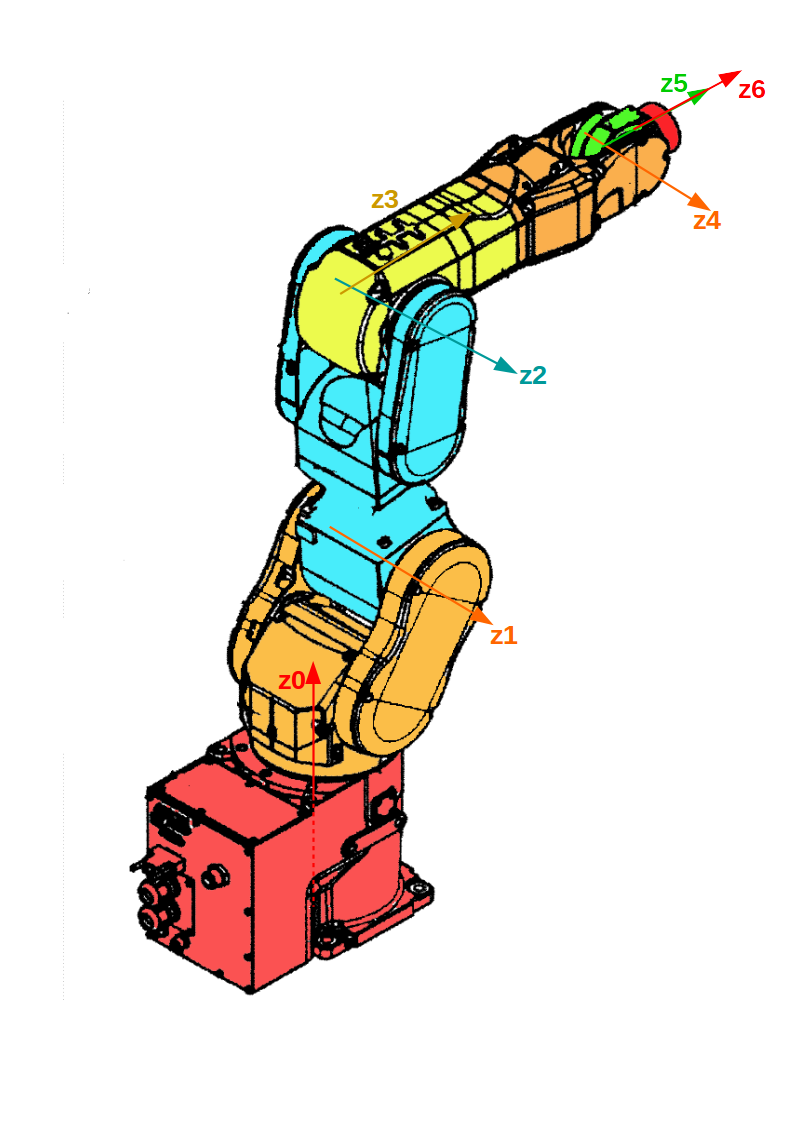以这张图（来源）为例，标准DH方法是在传动轴上（远端，如图）建立坐标系的，所以 z 轴（为了避免麻烦，只画z轴）建立在连杆远离基座一侧的那个轴上；改进DH方法则会把坐标系建立在固定轴上（近端）。
这两种建系方法对于平常的普通机械臂是没有区别的，在matlab里的体现也只是修改“modified”参数的问题，最多是得到的转换矩阵结果不同。但是对于delta、Atrias这样的并联机器人，或者树状、轮式结构机器人来说，在进行逆运算时，如果回推原点大于1个，就会出现混乱。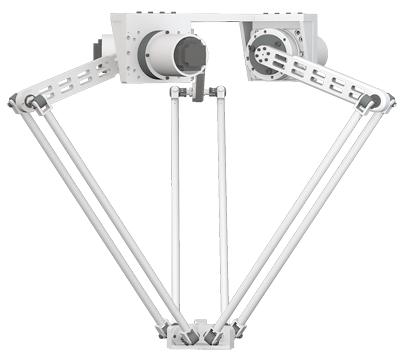delta机器人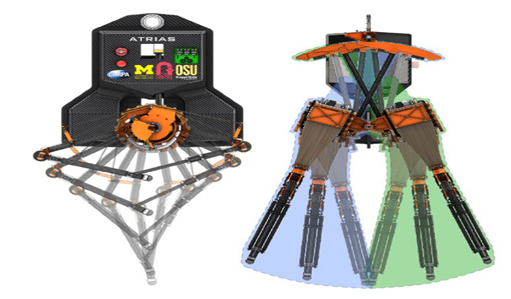Atrias机器人
展开全文开发语言
• 从晚上找过很多机器人工具箱，大多不好用，这个是好用的，已经验证过。包括matlab机器人工具箱和使用说明及一些例子。
• matlab机器人工具箱10.2，全新的机器人工具箱，内容更全，适合matlab的机器人仿真！ Matlab上用于机器人仿真非常好用的工具包，欢迎下载！
• matlab机器人工具箱包含两自由度和六自由度两种机器人的几种经典控制算法，只需在算法参数设置修改成自己的机器人结构参数，便可得到仿真的结果，也可在经典算法的基础上修改设计自己的算法。
• MATLAB工具箱大全- 机器人工具箱robot-10.3.1
• matlab 机器人工具箱 适合于毕业设计、自学、科研等 在MATLAB的环境下，使用RoboticsToolbox工具箱建立该机器人的数学模型，并对其进行运动学仿真及轨迹规划。 在官网下载的 绝对好用 安装路径 只需要在matlab ...
• 工具箱添加到matlab的方法： >>addpath RVCDIR >>startup_rvc RVCDIR是解压该文件夹之后，rvctools文件夹子文件所在目录，注意目录要到rvctools层。命令startup_rvc会将各种子文件添加给你的路径。
• 1、机器人工具箱安装 2、使用机器人工具箱之前，不需要每次都得输入startup_rvc

1、将名字为 rvctools 的文件夹（也就是你下载的机器人工具箱）复制到matlab的toolbox文件夹里

2、在matlab的设置路径里添加rvctools文件夹。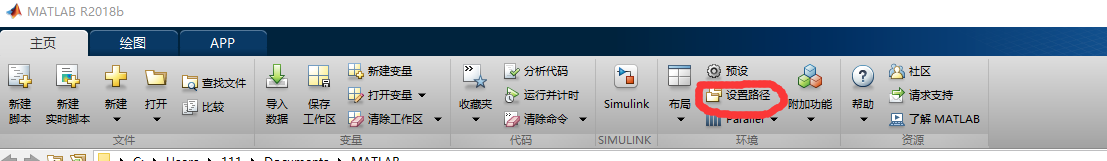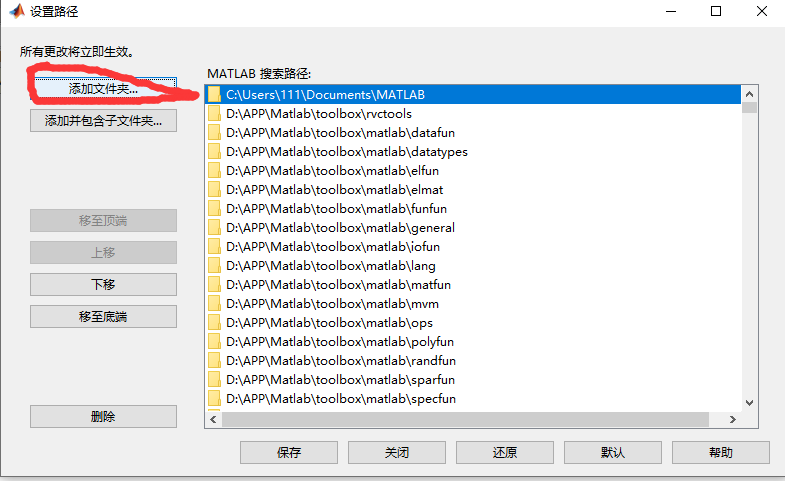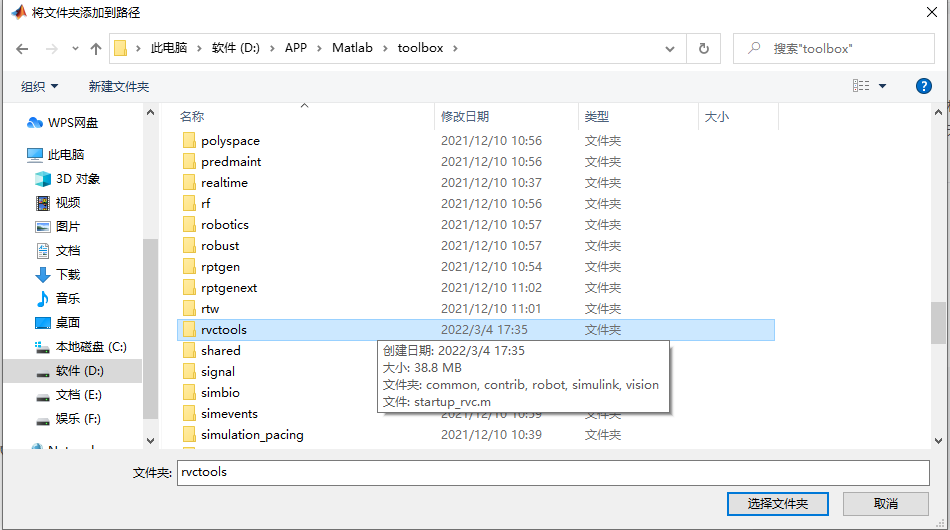④添加完毕之后，记得点保存

⑤  在matlab的命令行中输入startup_rvc,这样你就可以使用机器人工具箱了（每次使用机器人工具箱都需要输入一次）

⑥如果不想每次都得输入，你可以在MATLAB里新创建一个名为startup.m的文件，在里面输入两行代码

startup_rvc
clc

保存在toolbox文件夹里的local文件夹里，这样，每次启动matlab，就会自动运行这两行代码了

展开全文• 搜集到目前为止的四个版本，分别是8.0、9.8、9.10、10.2。再也不用担心命令不兼容了！
• matlab机器人工具箱的安装

# 学习目标：

matlab机器人工具箱的安装

# 学习内容：

1、 matlab机器人工具箱的安装

# 学习记录：

2.找到下载位置，如图所示：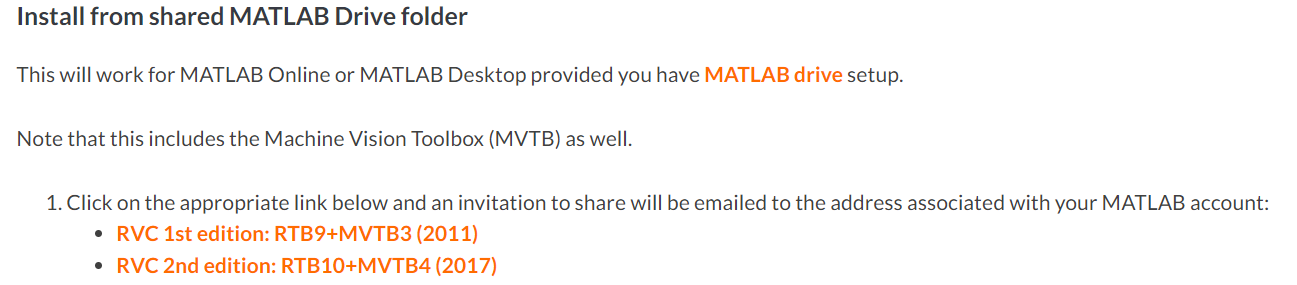3.下载rvctools文件包，放在一个自己知道的目录下(任意路径都是可以的，但是为了方便管理，一般都安装在toolbox)，然后“设置路径”里面添加并保存。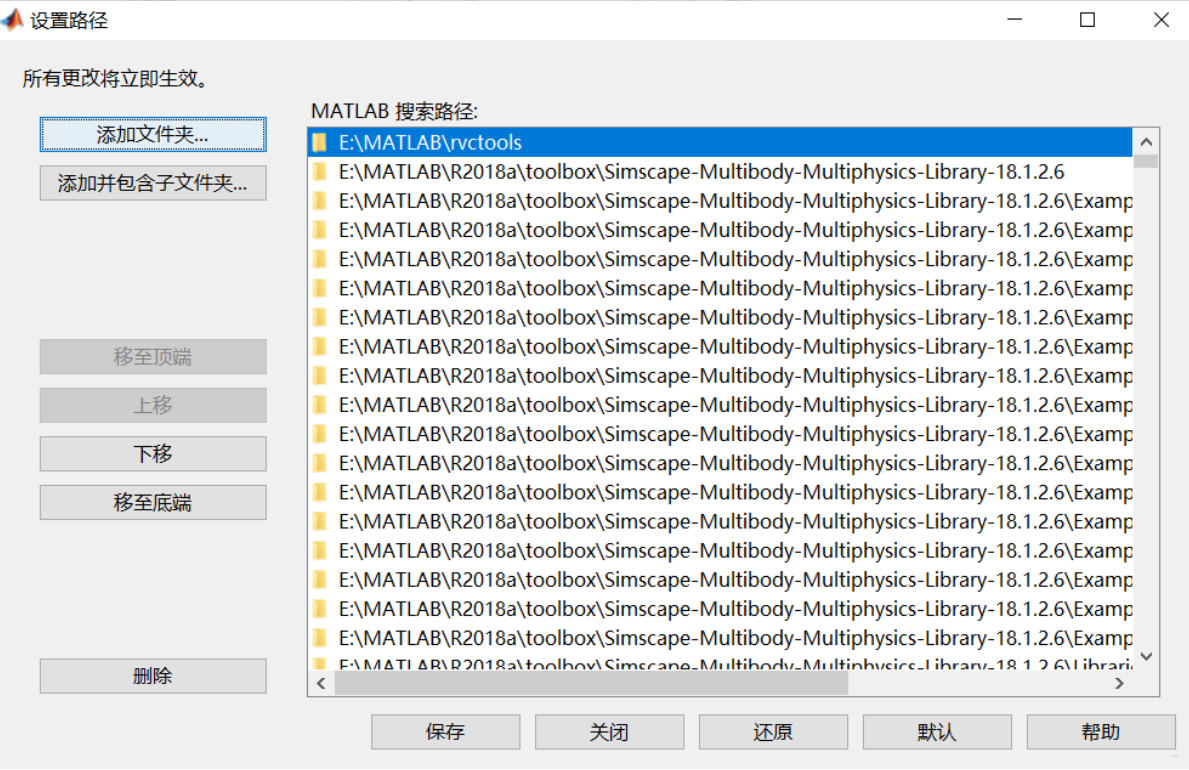4.在命令中输入startup_rvc，之后会看到机器人工具箱启动成功。
5.最后在命令行中输入rtbdemo命令。就可以看到操作界面。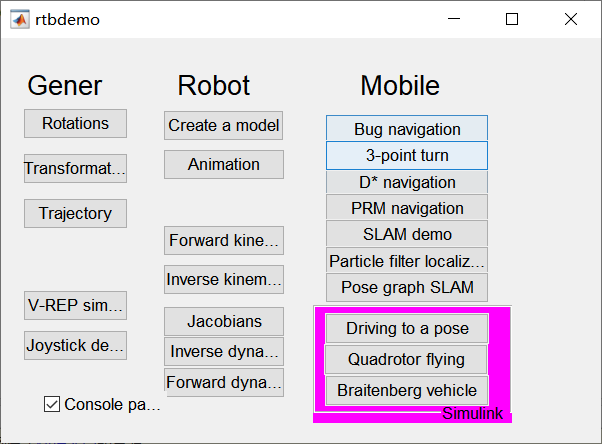展开全文• matlab机器人工具箱的使用（4） 学习内容： 1、工作空间可视化 学习记录： 思路： 随机生成一个关节变量-----fkine------>变换矩阵------transl--------->三维坐标 rand函数 rand是在（0，1）内生成一个...
• 学习目标： matlab机器人工具箱的使用（5） 学习内容： 1、工作空间可视化 学习记录：
• MATLAB机器人工具箱下载连接 机器人工具箱的安装 今天用到机器人工具箱，顺便在这里给大家分享一下，需要的可以参考哦！ 安装步骤： 解压下载完的机器人工具箱文件，将解压文件复制到 MATLAB 安装目录下的 ......

# matlab机器人工具箱matlab 订阅